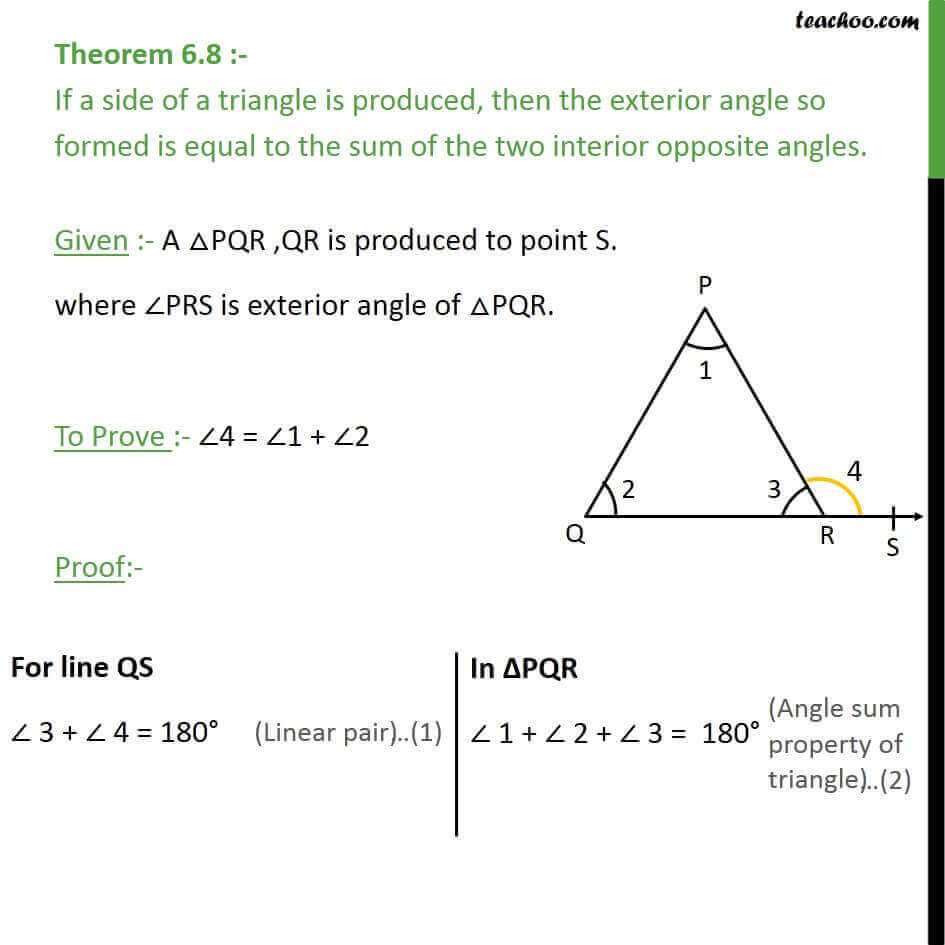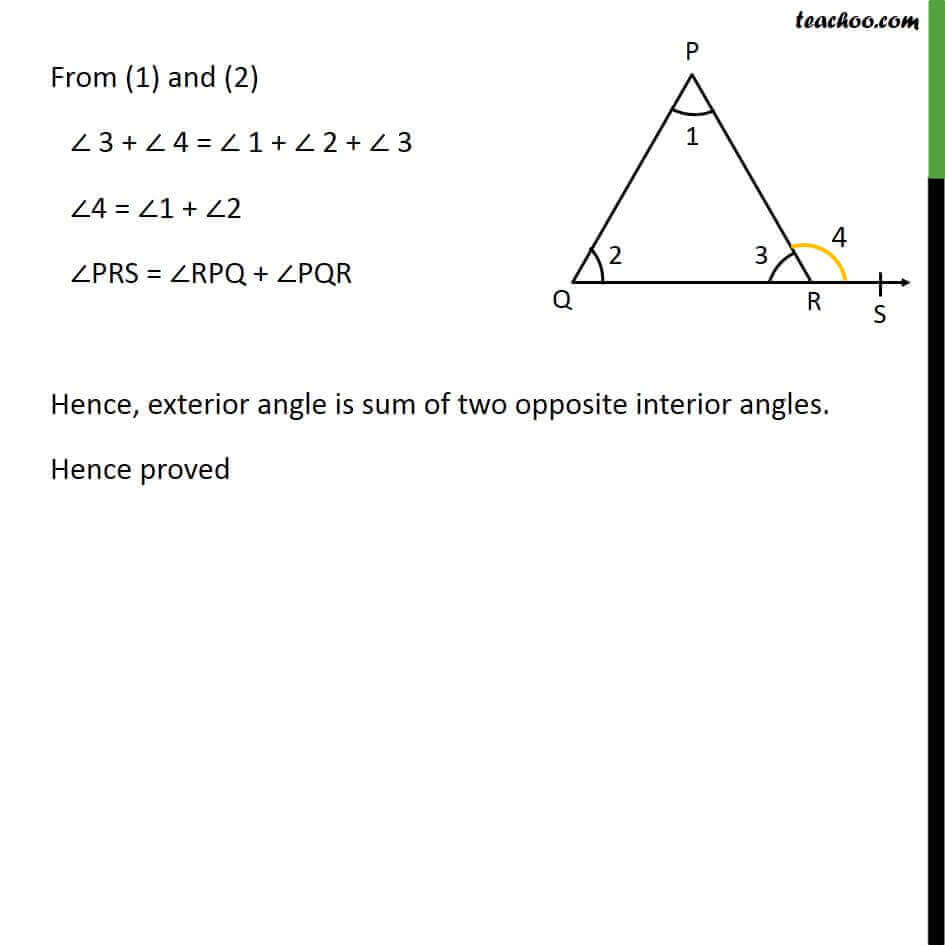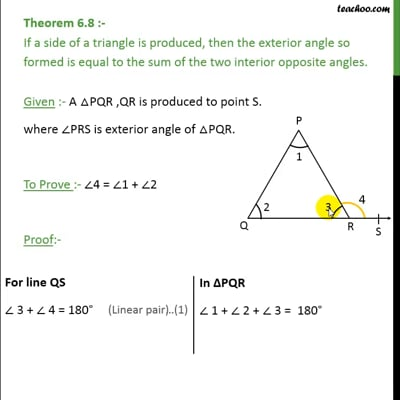Theorems

Chapter 6 Class 9 Lines and Angles (Term 1)
Serial order wiseThis video is only available for Teachoo black users

### Transcript

Theorem 6.8 :- If a side of a triangle is produced, then the exterior angle so formed is equal to the sum of the two interior opposite angles. Given :- A PQR ,QR is produced to point S. where PRS is exterior angle of PQR. To Prove :- 4 = 1 + 2 Proof:- From (1) and (2) 3 + 4 = 1 + 2 + 3 4 = 1 + 2 PRS = RPQ + PQR Hence, exterior angle is sum of two opposite interior angles. Hence proved